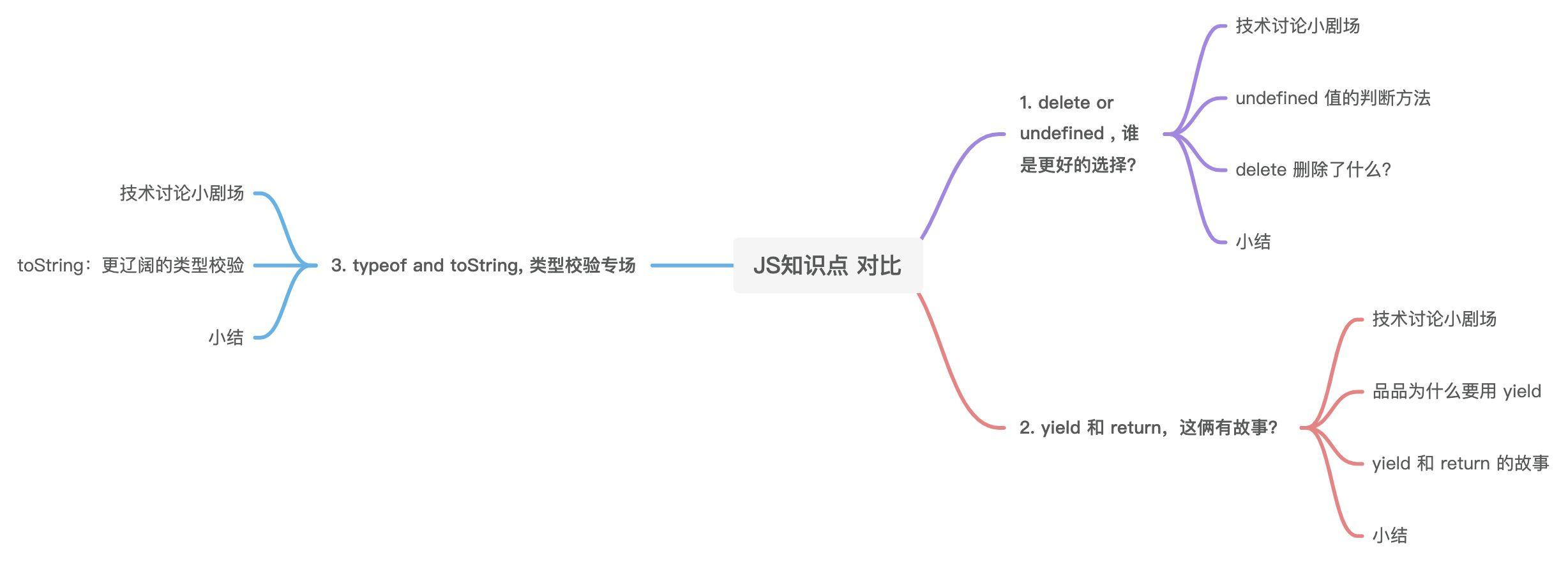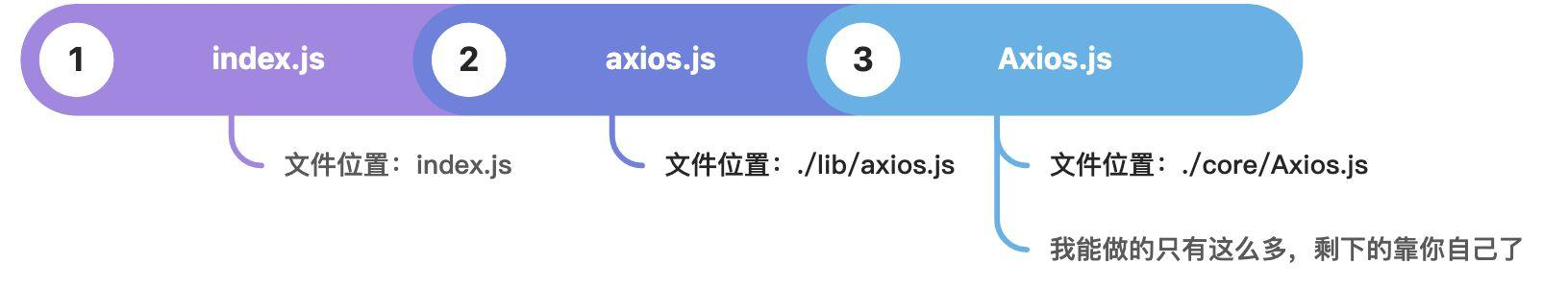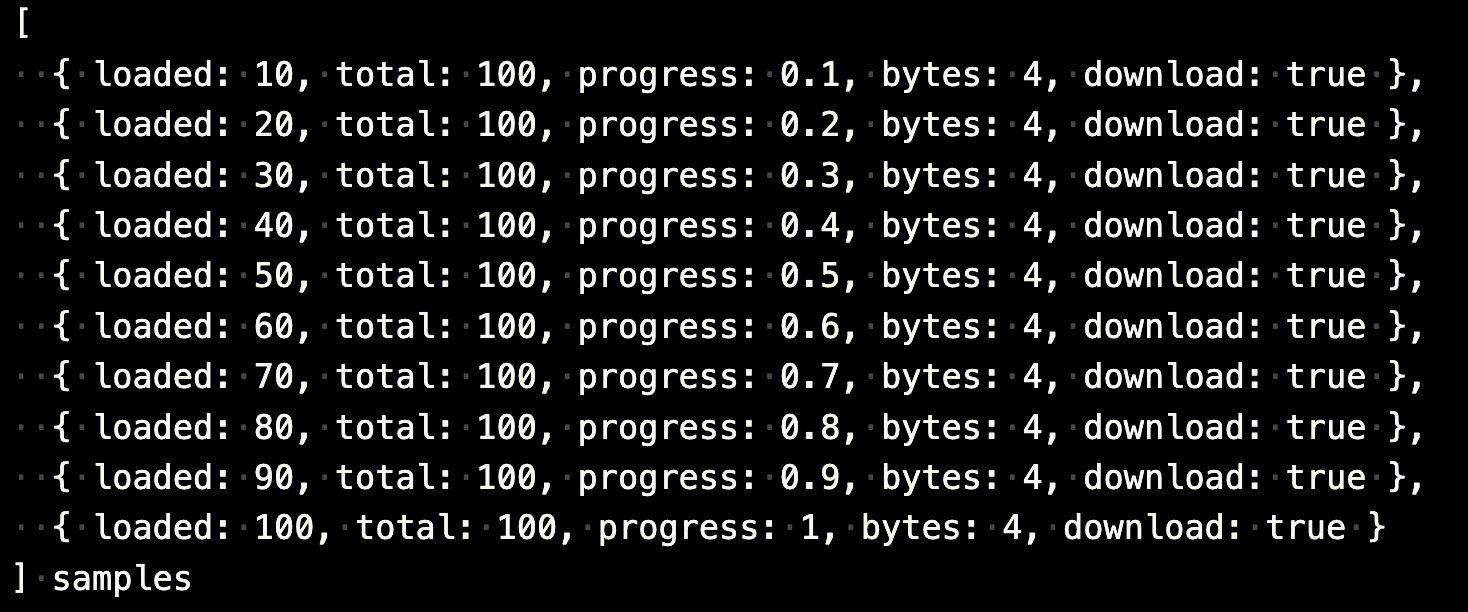# 文章速读# 知识点对比开始

## delete or undefined, 谁是更好的选择？

### undefined 值的判断方法

``````const typeOfTest = type => thing => typeof thing === type;

/**
* Determine if a value is undefined
*
* @param {*} val The value to test
*
* @returns {boolean} True if the value is undefined, otherwise false
*/
const isUndefined = typeOfTest('undefined');

const val = undefined;
const val2 = 2;
isUndefined(val) // true
isUndefined(val2) // false
``````

### delete 删除了什么？

#### 删除对象的某个属性

``````var obj = {
name: 'ye',
age: 18,
};
delete obj.age;
console.log(delete obj.age); // true
console.log(obj); // { name: 'ye' }
``````

#### 如果删除一个对象不存在的属性，会怎样？

``````var obj = {
name: 'ye',
age: 18,
};
delete obj.desc;
console.log(delete obj.desc); // true
console.log(obj); // { name: 'ye', age: 18 }
``````

#### delete ‘zhang’ or delete 18 结果会怎样？

``````console.log(delete 'zhang'); // true
console.log(delete 18); // true
``````

#### 删除 let 或 const 声明的属性会怎样？

``````var name = 'ye';
or
var name = obj.name;
console.log(delete name); // false
``````

### 小结

1. 不需要某个对象属性时，推荐将对象的值设置为 undefined，而不是delete 它。
2. 经过一系列实验会发现，delete 其实删除的是一个表达式的引用类型的结果。
3. axios 源码内容虽然不是特别多，但是完整的梳理清楚还是挺耗时的。我帮大家画个入口示意图，感兴趣的可以下载到本地，慢慢赏析。☞axios github## yield 和 return，这俩有故事？

### 品品为什么要用 yield

axios 源码里面有一段代码，在测试用例里面，实际的代码无法直接打印，我稍作了调整，如下：

``````const count = 10;
const chunkLength = 10;
const contentLength = count * chunkLength;
const samples = Array.from(
(function* () {
for (let i = 1; i <= 10; i++) {
yield {
total: contentLength,
progress: (chunkLength * i) / contentLength,
bytes: 4,
};
}
})(),
);
````````````console.log(samples); // []
``````

Array.from 支持通过伪数组对象创建数组对象。伪数组对象是指拥有一个 length 属性和若干索引属性的任意对象。

``````const count = 10;
const chunkLength = 10;
const contentLength = count * chunkLength;
const samples = Array.from({ length: count }, (_, j) => {
let i = j + 1;
return {
total: contentLength,
progress: (chunkLength * i) / contentLength,
bytes: 4,
};
});
``````

### yield 和 return 的故事

#### 相同场景

``````function* foo(index) {
while (index < 2) {
index++;
yield index;
}
}

const iterator = foo(0);

console.log(iterator.next().value); // 1
``````

``````function* foo(index) {
while (index < 2) {
index++;
return index;
}
}

const iterator = foo(0);

console.log(iterator.next().value); // 1
``````

#### 如果把返回的这行代码提升会怎么样？

``````function* foo(index) {
while (index < 2) {
yield index;
index++;
}
}

const iterator = foo(0);

console.log(iterator.next().value); // 0
console.log(iterator.next().value); // 1
``````

``````function* foo(index) {
while (index < 2) {
return index;
index++;
}
}

const iterator = foo(0);

console.log(iterator.next().value); // 0
console.log(iterator.next().value); // undefined
``````

• return 语句终止函数的执行。
• yield 语句挂起当前函数，而下一次调用 next() 时，在 yield 之后紧接着的语句会继续执行

#### 听说 yield 能传值？

``````function* func(x) {
console.log('x:', x);
x--;
x = yield x;
}
let generatorFunc = func(10);
let value = generatorFunc.next().value;
console.log('value:', value);
``````

``````x: 10 // 第一个console
value: 9 // 第二个console
``````

### 小结

1. yield 关键字用于暂停和恢复生成器函数。
2. yield 和 return 的故事在于，yield可以被认为是一个基于生成器的版本的 return 关键字。但是 yield 功能更丰富。
3. axios 源码，看到哪里算哪里吧。

## typeof and toString, 类型校验专场

### toString：更辽阔的类型校验

``````const { toString } = Object.prototype;
const kindOf = (cache => thing => {
const str = toString.call(thing);
return cache[str] || (cache[str] = str.slice(8, -1).toLowerCase());
})(Object.create(null));

const kindOfTest = type => {
type = type.toLowerCase();
return thing => kindOf(thing) === type;
};
/**
* Determine if a value is a Date
*
* @param {*} val The value to test
*
* @returns {boolean} True if value is a Date, otherwise false
*/
const isDate = kindOfTest('Date');

console.log(isDate(new Date())); // true
console.log(isDate('ye')); // false
``````

``````console.log(Object.prototype.toString.call(111)); // [object Number]
console.log(Object.prototype.toString.call('ye')); // [object String]
console.log(Object.prototype.toString.call(true)); // [object Boolean]
console.log(Object.prototype.toString.call(null)); // [object Null]
console.log(Object.prototype.toString.call(undefined)); // [object ReUndefinedgExp]
console.log(Object.prototype.toString.call(Symbol())); // [object Symbol]
console.log(Object.prototype.toString.call([])); // [object Array]
console.log(Object.prototype.toString.call(function () {})); // [object Function]
console.log(Object.prototype.toString.call(new Error())); // [object Error]
console.log(Object.prototype.toString.call(new Date())); // [object Date]
console.log(Object.prototype.toString.call(new RegExp())); // [object RegExp]
console.log(Object.prototype.toString.call(Map)); // [object Function]
console.log(Object.prototype.toString.call(Math)); // [object Math]
``````

### 小结

1. Object.prototype.toString.call() 几乎可以实现所有数据类型的校验。
2. 想要获取准确的数据类型，还要在 [object Type] 的基础上通过正则匹配获取最终的 Type。
3. 方法都是现成的，需要哪个用哪个。掌声送给 axios 源码。

# 总结